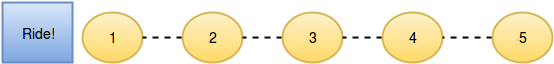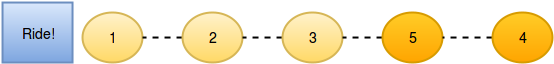# Riddle: count the moves

• i have this set of numbers:

2 1 5 3 4
5 jumpt over 4
5 jumpt over 3
2 jumpt over 1

in total there where 3 moves ( witch is the expected result)
these pictures represent the expected result in the order they ...next set of number:
12537864

here there expected result is: 7
5 jumps 4
5 jumps 3
7 jumps 6
7 jumps 4
8 jumps 6
8 jumps 4
6 jumps 4

this is the code i have sofar

``````bool isConsecutiveToLast(vector<int> &q, int i, int &size){
bool result = false;
if((i > 0) && (i < size-1)){
int lower = q.at(i-1) +1;
int mid = q.at(i);
if (lower == mid){
//std::cout << "consecutive: " << q.at(i) << " " << q.at(i+1)<< std::endl;
result = true;
}
else{
result = false;
}
}
else{
result = false;
}
return result;
}

bool noMove(vector<int> &q, int i, int &size){
bool result = false;
if(q.at(i) == (i + 1)){
//std::cout << q.at(i) << " no move" << std::endl;
result = true;
}
else{
result = false;
}
return result;
}

bool hasMoved(vector<int> &q, int i, int &size){
bool result = false;
if(q.at(i) != (i + 1)){
//std::cout << q.at(i) << " result: " << std::abs(q.at(i) - (i + 1)) << std::endl;
result = true;
}
else{
result = false;
}
return result;
}

void minimumMoves(vector<int> q) {
for(auto ii : q){
std::cout << ii;
}
std::cout << std::endl;
std::cout << "-----------------" << std::endl;
std::cout << q.size() << std::endl;
std::cout << "-----------------" << std::endl;

int i = 0, j = 0, size = q.size();
int counter = 0;
int counterConsecutive = 0;
bool consecutiveBool = false;
for(i = 0; i < size ; i++){

if(noMove(q, i, size)){
std::cout << q.at(i) << " no move" << std::endl;
}

if(hasMoved(q, i, size)){
int temp = std::abs((q.at(i) - (i + 1)));
std::cout << q.at(i) << " has moved: "<<  temp << " Spots" << std::endl;
}

if(isConsecutiveToLast(q, i, size)){
std::cout << "consecutive: " << std::endl;
}
}
std::cout << std::endl;
std::cout << "-----------------" << std::endl;
std::cout << "counter: " << counter - counterConsecutive << std::endl;
}

``````

this is what my code returns for the first set of numbers, going forward instead backwards.

``````21534

-----------------

5

-----------------

2 has moved: 1 Spots

1 has moved: 1 Spots

5 has moved: 2 Spots

3 has moved: 1 Spots

4 has moved: 1 Spots
``````

for the other set of Number:

``````12537864

-----------------

8

-----------------

1 no move

2 no move

consecutive:

5 has moved: 2 Spots

3 has moved: 1 Spots

7 has moved: 2 Spots

8 has moved: 2 Spots

consecutive:

6 has moved: 1 Spots

4 has moved: 4 Spots
``````

i have been coding for hours, but ....

who can help?

• I might be a little dense in the evening but I'm not sure what the rules are.
Do I understand correctly that you're just sorting and trying to count the number of swaps? Your first example sorta looks like bubble sort with a counter.

• Indeed it looks like bubble sort but with an added twist of keeping track of the no. of bubbles, maybe something like this (a simple console program):

``````#include <QCoreApplication>

int main(int argc, char *argv[])
{
QCoreApplication a(argc, argv);

auto qputs = [](QString s) { puts(qUtf8Printable(s)); };
//    QVector<int> v = {2,1,5,3,4};
QVector<int> v = {1,2,5,3,7,8,6,4};

for (int i = 0; (i < v.size()); ++i)
for (int j = v.size() - 1; (j > i); --j)
if (v[i] > v[j])
{
for (int s = i; (s < j); ++s)
v.swapItemsAt(s,s + 1);

qputs(QString::number(v[j]) + " has moved: " + QString::number(j - i) + " spots.");
}
}
``````

Note: I changed from using std::vector to QVector, this is a Qt forum after all :-)

• @Chris-Kawa

hmmmm!

i didn't know that

https://www.geeksforgeeks.org/bubble-sort/

this might apply, not sure.

• I know this is probably a homework or something, but it's been probably close to two decades since I last implemented bubble sort so why not :)

Printing:

``````void print(const std::vector<int>& v)
{
for (int val : v)
std::cout << val << " ";
std::cout << "\n";
}
``````

swapping with outputing the move:

``````void swap(int& a, int& b)
{
std::cout << a << " jumps " << b << "\n";
int temp = a;
a = b;
b = temp;
}
``````

sorting:

``````void bubbleSort(std::vector<int>& v)
{
for (int i = v.size() - 1; i > 0; --i)
{
for (int j = 0; j < i; ++j)
{
if (v[j] > v[j + 1])
swap(v[j], v[j+1]);
}
}
}
``````

and then use:

``````std::vector<int> v {1,2,5,3,7,8,6,4};

print(v);
bubbleSort(v);
print(v);
``````

Output:

``````1 2 5 3 7 8 6 4
5 jumps 3
8 jumps 6
8 jumps 4
7 jumps 6
7 jumps 4
6 jumps 4
5 jumps 4
1 2 3 4 5 6 7 8
``````

• thnx

ya, that's the difference between someone that has or had computer science teacher could ask questions and someone that is doing this on his own.

30 days ago, i started @ the 2.2million+ position and currently I'm ranked at slightly under 200.000.
the solution was probably in their discussion board on the topic, but that the same as cheating.
so i insist on doing it on my own, but this one ... beat me.

i also codec a Midi sequencer in a Pic18F452, acurate to 999BPM, with a linked list, function pointers in C.
but i never got a programming job.

• I also never had a computer science teacher, I learned it by myself. It is a slower way, but it can work for you also, just keep at it!

• @hskoglund

they problem is how to convince someone?
they want degrees, no matter how good you're or bad!

even though you have been playing with computers since 1993 and forced Microsoft to change Windows Task Manager.

hopefully this will help.

2 bad it's currently so hot, because normally i would solve like 2/3 of these riddle a day.
although they are getting harder.

• ya, that's the difference between someone that has or had computer science teacher could ask questions and someone that is doing this on his own.

I don't think you need a teacher or a class for this. Bubble sort is literally somewhere in the first 5 things every programming tutorial/video/book/magazine article does right after hello world.
My honestly brutal and divisive opinion on formal programming education is that it only gives you a bunch of keywords that you can follow up on your own, so it maybe cuts the first step in some cases. The sad truth is most people straight out of school are useless and have theoretical knowledge that is often harmful because thinking that you know something is far worse than knowing and admitting you don't know :)
As for a degree - I never worked at a place that paid any attention to that. Honestly if an interview started with that I would have walked out because it gives an idea of priorities at that place. But that's just the comfort of the niche I work in I guess. There are lines of work that simply require a degree because of regulations or such, but if you can't get into that there's a lot of programming job lines that value an actual skill over a piece of paper with a stamp.

• This post is deleted!

• Hey, in today's world a "social media influencer" is an actual job title and often paid better than technical positions ;)
What can you do. Times they are a-changin', adapt or get left behind.

i have a Book on:
Perl
Java
Java2
programming in C# 3.0
but i didn't know that expression (bubble sort).

I have an equally controversial opinion on all these languages and what they did to the industry, but I feel I'm already being too negative, so I'll restrain myself from voicing that opinion ;) All I can say is if a book on a programming language doesn't mention basic algorithms then there's probably a better book out there. I'd say you would be far better to read a book on algorithms and data structures, which are universal across technologies, instead of another language, because that's just slightly different syntax solving the same basic problems - data relations, finding, indexing, structured traversal, sorting, data access patterns, etc.

• thnx, it does indeed work in the required or expected steps, but i also need to keep track of the swap distance.
so i had to modify the swap function.

``````void swap(vector< pair <int, int> > &data, int& a, int& b)
{
if (std::find(data.begin(), data.end(), std::pair<std::int, std::int>(a, 0)) != data.end()){ // Zero is problem
for(auto ii : data){
if(ii.first == a){
ii.second = ii.second +1;
}
}
}
else{
data.push_back( make_pair(a, 0) );
}

std::cout << a << " jumps " << b << "\n";
int temp = a;
a = b;
b = temp;
}
``````

error:

``````Solution.cpp:16:73: error: wrong number of template arguments (1, should be 2)
``````

i know only the first, if not present in the vector add, otherwise update it.

regards

• This post is deleted!

• @Natural_Bugger said:

so i had to modify the swap function.

I think you've overcomplicated this. Taking a step back and looking at the problem - you want to count number of jumps for given number. Vector of pairs can work but a far simpler structure for this is a map. The key would be the number and the value is the amount of swaps it did, e.g.

``````void swap(std::map<int, int>& counts, int& a, int& b)
{
counts[a]++;
std::cout << a << " jumps " << b << "\n";
int temp = a;
a = b;
b = temp;
}
``````

Then you can print it like this:

``````for (auto& c : counts)
std::cout << "Number " << c.first << " jumped " << c.second << " time(s)\n";
``````

If you want to count both the number jumping and being jumped you can add `counts[b]++` in there too. Or you could count those cases in separate maps, whatever your need is.

• @Chris-K
thnx,

that works ... some minor things to do and done!

• @Chris-Kawa

sometimes you can oversee the simple things.
counts[a]++;

• @Chris-Kawa

the explication of some of the riddles there is written complicatedly.
as in this.

: )

• @Chris-Kawa

ha, the code is good, but does not pas all tests.
it doesn't pass the tests within in the time limit, handling set of numbers up to 100000.

``````Your code did not execute within the time limits
``````
``````
void swap(std::map<int, int>& countsdata, int &count, int& a, int& b)
{
countsdata[a]++;
int temp = a;
a = b;
b = temp;
count++;
}

void minimumMoves(vector<int> q) {
std::map<int, int> countsdata;
int count = 0;

for (int i = q.size() - 1; i > 0; --i)
{
for (int j = 0; j < i; ++j)
{
if (q[j] > q[j + 1])
swap(countsdata, count, q[j], q[j+1]);
}
}

bool tooChoatic = false;
for (auto& c : countsdata){ // i suspect this is the culprit.
if(c.second > 2){
tooChoatic = true;
break;
}
}

if(tooChoatic){
std::cout << "Too chaotic" << std::endl; // jumped more than 2 positions.
}
else{
std::cout << count << std::endl;
}
}
``````